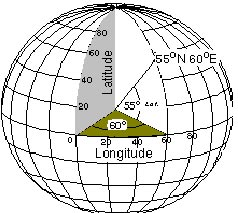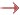Home    |    Concepts   |   API   |   Samples
Concepts > Geometry > Coordinate References > Coordinate Systems
Geographic Coordinate SystemsA geographic coordinate system is a three-dimensional reference system that locates points on the Earth's surface. The unit of measure is usually decimal degrees. A point has two coordinate values: latitude and longitude. Latitude and longitude measure angles.

Latitude is defined as the angle formed by the intersection of a line perpendicular to the Earth's surface at a point and the plane of the Equator. Points north of the Equator have positive latitude values, while points south have negative values. Latitude values range from -90 to +90 degrees. Lines of latitude are also called parallels because a particular value of latitude forms a circle parallel to the Equator.

A meridian, or line of longitude, is formed by a plane that passes through the point and the North and South poles. The longitude value is defined by the angle between that plane and a reference plane. The reference plane is known as the prime meridian. The most common prime meridian passes through Greenwich, United Kingdom. Other examples of prime meridians in use pass through Paris and Bogot Longitude values range from -180 to +180 degrees.

Even though geographic coordinates are angular units, ArcSDE stores and treats them as if they are planar. In this case, longitude values are considered the x-coordinate, while latitude values are the y-coordinate.

A geographic coordinate system has the following components:

• Angular units: The unit of measure on the spherical reference system.
• Spheroid: The reference spheroid for the coordinate transformation.
• Datum: Defines the relationship of the reference spheroid to the Earth's surface.
• Prime meridian: The longitude origin of the spherical reference system.

The following example is a string representing a geographic coordinate system based on the WGS 1984 datum.

 GEOGCS["GCS_WGS_1984",DATUM["D_WGS_1984",SPHEROID["WGS_1984",6378137, 298.257223563]],PRIMEM["Greenwich",0],UNIT["Degree",0.0174532925199433]]

The coordinate system for a layer is stored in the LAYERS table as a text string that may be as long as 1024 characters.Geographic Transformation MethodsNational Transformation version 2Defining a Geographic (Datum) TransformationUsing Grid-based Geographic Transformations